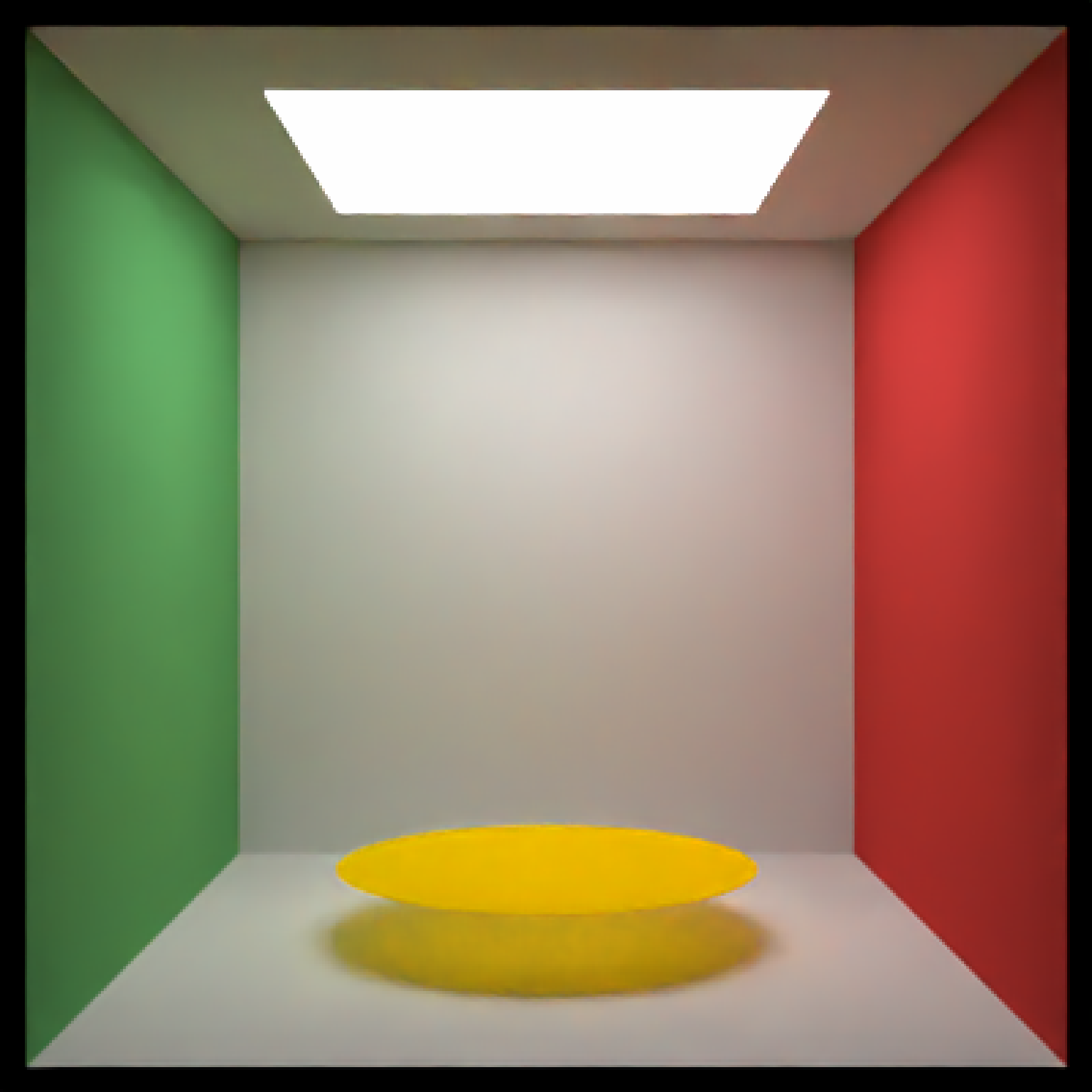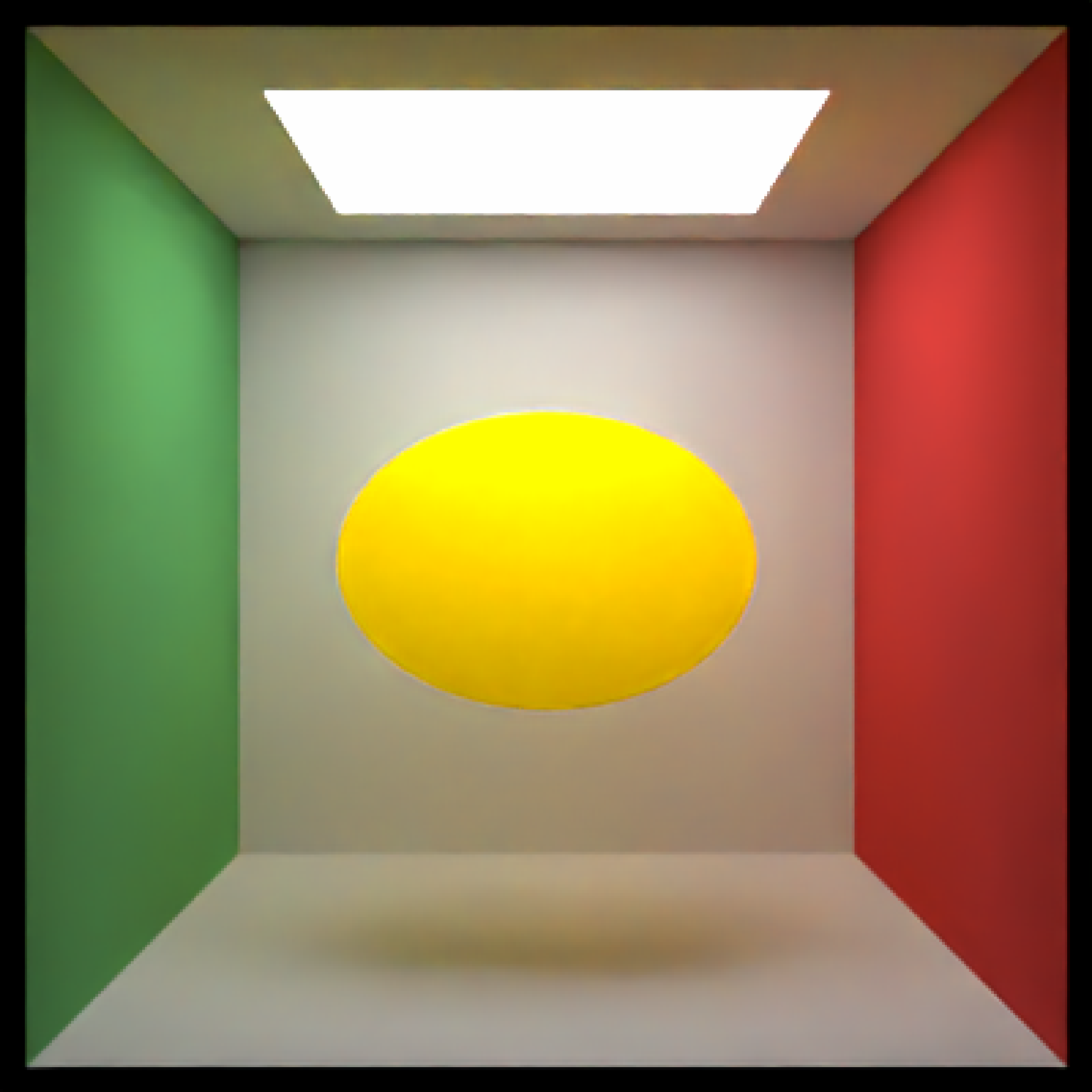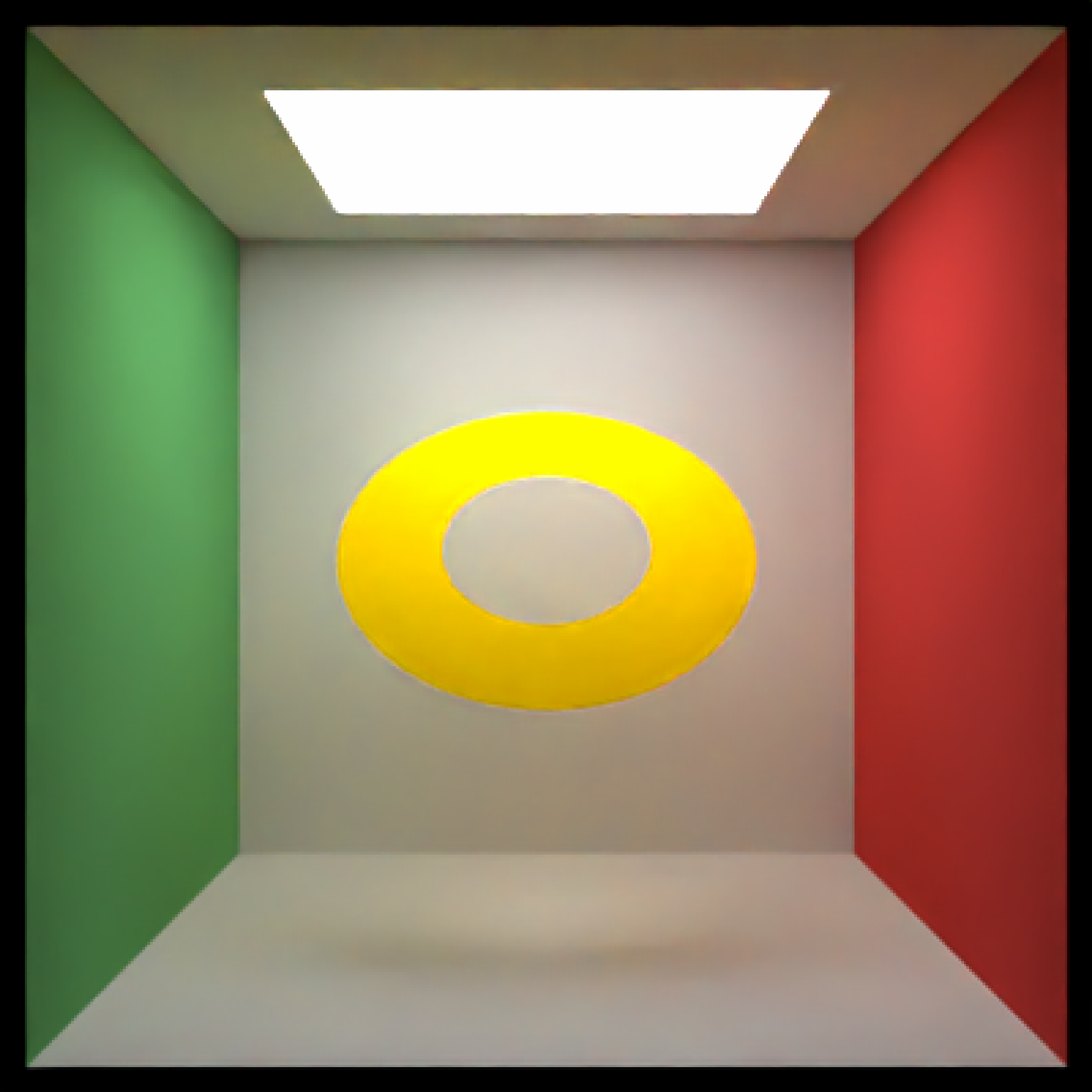Disk Object

disk(
x = 0,
y = 0,
z = 0,
material = diffuse(),
angle = c(0, 0, 0),
order_rotation = c(1, 2, 3),
velocity = c(0, 0, 0),
flipped = FALSE,
scale = c(1, 1, 1)
)

## Arguments

x Default 0. x-coordinate of the center of the disk Default 0. y-coordinate of the center of the disk Default 0. z-coordinate of the center of the disk Default 1. Radius of the disk. Default 0. Inner radius of the disk. Default diffuse.The material, called from one of the material functions diffuse, metal, or dielectric. Default c(0, 0, 0). Angle of rotation around the x, y, and z axes, applied in the order specified in order_rotation. Default c(1, 2, 3). The order to apply the rotations, referring to "x", "y", and "z". Default c(0, 0, 0). Velocity of the disk. Default FALSE. Whether to flip the normals. Default c(1, 1, 1). Scale transformation in the x, y, and z directions. If this is a single value, number, the object will be scaled uniformly. Note: emissive objects may not currently function correctly when scaled.

## Value

Single row of a tibble describing the disk in the scene.

## Examples

#Generate a disk in the cornell box.
# \donttest{
generate_cornell() %>%
add_object(disk(x = 555/2, y = 50, z = 555/2, radius = 150,
material = diffuse(color = "orange"))) %>%
render_scene(lookfrom = c(278, 278, -800) ,lookat = c(278, 278, 0), fov = 40,
ambient_light = FALSE, samples = 400, parallel = TRUE, clamp_value = 5)# }
#Rotate the disk.
# \donttest{
generate_cornell() %>%
add_object(disk(x = 555/2, y = 555/2, z = 555/2, radius = 150, angle = c(45, 0, 0),
material = diffuse(color = "orange"))) %>%
render_scene(lookfrom = c(278, 278, -800) , lookat = c(278, 278, 0), fov = 40,
ambient_light = FALSE, samples = 400, parallel = TRUE, clamp_value = 5)# }
#Pass a value for the inner radius.
# \donttest{
generate_cornell() %>%
add_object(disk(x = 555/2, y = 555/2, z = 555/2,
ambient_light = FALSE, samples = 400, parallel = TRUE, clamp_value = 5)# }